# Chi-Square Critical Value – Excel and Google Sheets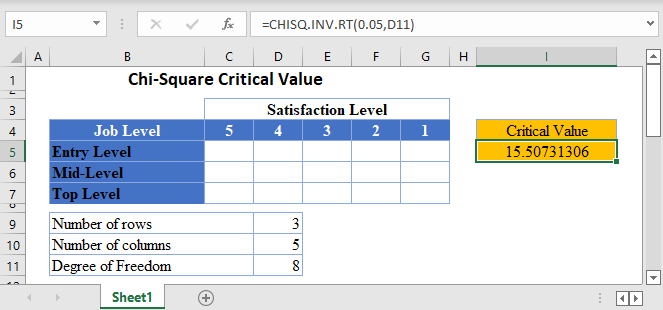Chi-Square (χ^2) test is a statistical test used to determine whether observed data from a sample matches the theoretical (expected) data from the population, or whether there is a relationship (association) between two variables.

The Chi-Square critical value is the value at which the Chi-Square test reports a result that is less probable than a pre-determined probability, called the significance level α .

When you perform a Chi-Square Test of Independence of Goodness of Fit Test, you obtain a test statistic, called Chi-Square observed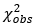, as a result. You then compare this test statistic with the Chi-Square critical value to determine whether the Chi-Square test is statistically significant or not.

## How to Find Chi-Square Critical Value in Excel

To obtain the Chi-Square critical value in Excel, you use the CHISQ.INV.RT Function.

### CHISQ.INV.RT Function

The CHISQ.INV.RT Function returns the inverse of the right-tailed probability (the critical value) of the chi-squared distribution which is used to make a conclusion whether a Chi-Square test is statistically significant or not.

If the observed Chi-Square value (test statistic) is greater than the critical value, it means that the Chi-Square test is statistically significant, and the null hypothesis is rejected. That is, the sample data does not match the expected data from the population for a goodness of fit test or there is an association/relationship between the variables in the dataset for a test of independence.

### CHISQ.INV.RT Function Syntax

``=CHISQ.INV.RT(probability, degree of freedom)``
• Probability: the probability value for the CHISQ.INV.RT Function is the significance level for which critical value you wish to obtain.
• The degree of freedom is calculated depending on the type of Chi-Square test being conducted. For the Chi-Square goodness of fit test, the degree of freedom is given by the number of observations, k, minus 1 (k-1). For the Chi-Square test of independence presented in a contingency (two-way) table,  the degree of freedom is given by the number of rows, r, minus 1 times the number of columns, c, minus 1; (r-1)(c-1).

### How to Use CHISQ.INV.RT Function – Test of Independence

Background: A statistician wants to investigate the relationship between job levels and job satisfaction for employees in a certain industry in the United States. He grouped employees into three categories; entry-level, mid-level, and top-level employees. A sample of employees from each category were asked to rate how satisfied they are with their current job roles based on a 5-point rating scale, 1 to 5, where 5 signifies very satisfied. Calculate the critical value for this test at a 5% significant level.

First, identify the number of rows and the number of columns. We visualize the arrangement of the result of the investigation in the following Excel table: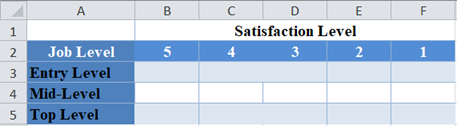From the above table, you can see that we have three rows (entry-level, mid-level, and top-level), and five columns (5, 4, 3, 2, and 1).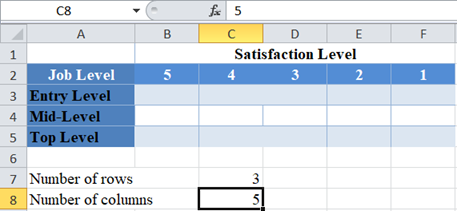Next, calculate the degree of freedom.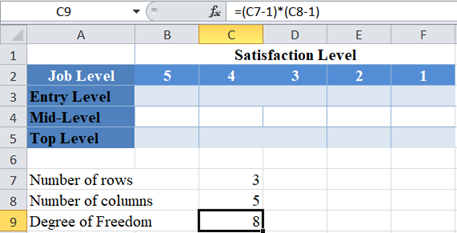Finally, calculate the required critical value using the CHISQ.INV.RT Function.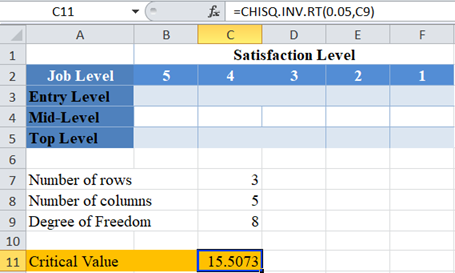Note that the probability is the significance level, which for our case is 5% or 0.05.

### The Chi-Square Critical Values Table

You can verify the above result using the standard Chi-Square table of critical values as shown below.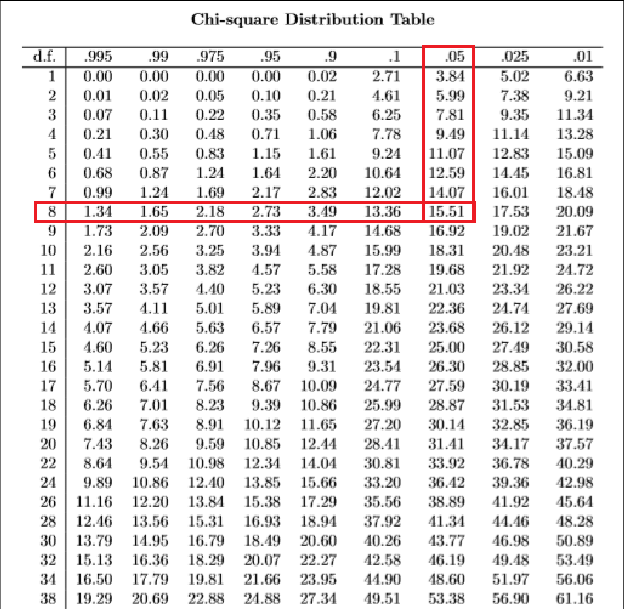### How to Use CHISQ.INV.RT Function – Goodness of Fit Test

Background: For the past five years, the average SAT test scores of candidates taking SAT from certain eight states has been approximately equal. A statistician wants to investigate whether the average test scores from these eight states in this year’s SAT follow the same pattern as the past five years. He conducts a Chi-Square test of the average test scores from this year’s SAT against the expected average test scores based on the last five years’ pattern and observed a Chi-Square value of . Calculate the critical value for this test at a 5% significant level, and hence determine whether the average test scores in this year’s SAT follow the same pattern as in the past five years for these eight states..

First, identify the number of observations. We visualize the data in the following Excel table: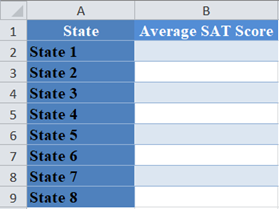From the above table, you can see that there are eight observations (average test scores from eight states).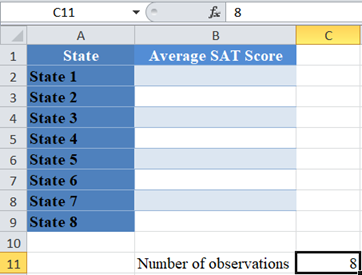Next, calculate the degree of freedom. Recall that the degree of freedom for the Chi-Square goodness of fit test is given by the number of observations minus 1.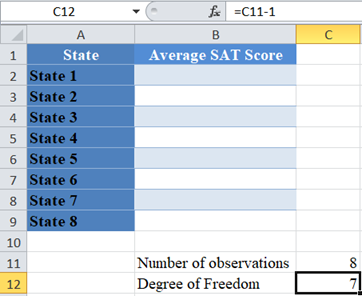Finally, calculate the required critical value using the CHISQ.INV.RT Function.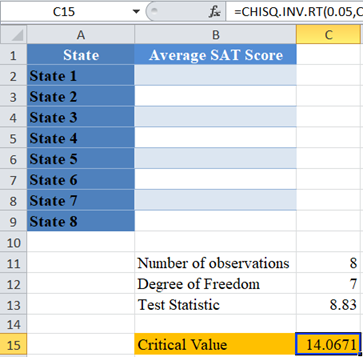Note that the probability is the significance level, which for our case is 5% or 0.05.

### Easy Excel Automation### The Chi-Square Critical Values Table

You can verify the above result using the standard Chi-Square table of critical values as shown below.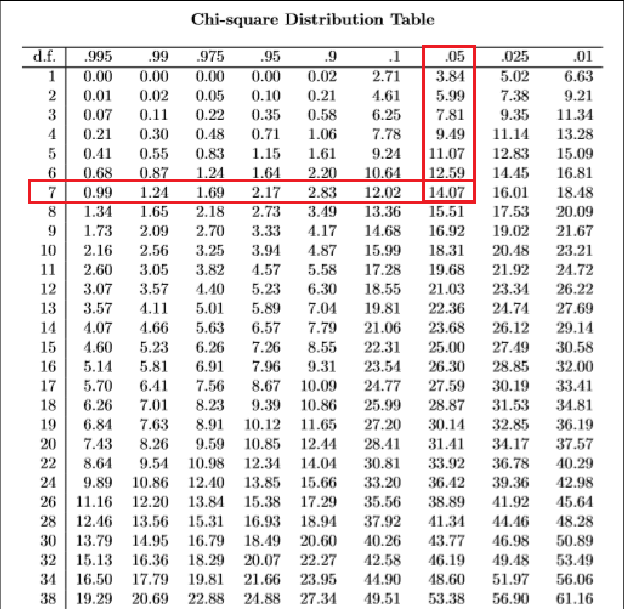Here, the observed Chi-Square (test statistic) is 8.83 which is less than the critical value of 14.0671. Hence, the Chi-Square test is not significant, and you “fail to reject the null hypothesis”. That is, there is not enough statistically significant evidence to suggest that the average test scores in this year’s SAT do not follow the same pattern as in the past eight years for these eight states.

## How to Find Chi-Square Critical Value in Google Sheets

The Chi-Square critical level can be calculated using a similar method in Google Sheets.

You can use the CHISQ.INV.RT Function the same way as in Excel.

The Chi-Square test of independence critical value in Google Sheets is as follows: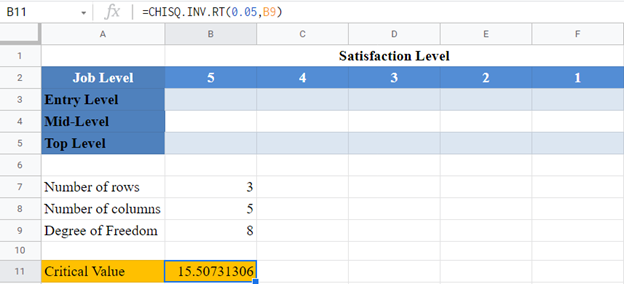And the Chi-Square goodness of fit test critical value in Google Sheets is as follows: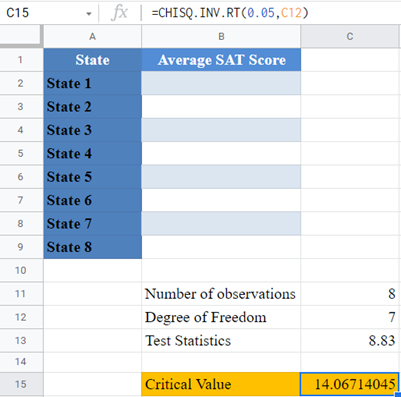## Common Errors –CHISQ.INV.RT Function

Some of the errors you may encounter when using the CHISQ.INV.RT Function.

• INV.RT Function returns #VALUE error value when either the probability or the degree of freedom arguments is not a number.
• INV.RT Function returns #NUM error value when:
• The probability is greater than 1 or less than 0.
• The degree of freedom is less than 1 or greater than .
• INV.RT Function expects the degree of freedom argument to be an integer, when not an integer, the function returns the value for the integer part. That is, CHISQ.INV.RT Function will return the same value for the degrees of freedom values of 3, 3.2, 3.8, etc.